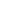Home   What's New   Market Research   Presentations### MONTE CARLO SIMULATION

Abukar M AliPlease note that this description and the accompanying spreadsheet are instructional tools, to be used for educational purposes only.

 We introduce here Monte Carlo simulation, as an introduction for users of the accompanying Excel spreadsheet application. The purpose of this workbook is to introduce the  numerical technique called Monte Carlo simulation, used  to price an equity call option. The technique can be extended to more complicated derivatives such as American call options and other path dependent options. A Monte Carlo simulation of a stochastic process is a procedure for sampling random outcomes for the process. The most common way to find the possible evolution of a stock by using the Monte Carlo simulation is by using the Geometric Brownian Motion we have already described. A path for the stock can be simulated by sampling repeatedly forfrom a standardized normal distribution. The Monte Carlo Simulation (MC) gives us the opportunity to see many different probable paths of the underlying security under the assumption that the returns of the instrument are normally distributed as the random variable we use is taken from a standardized normal distribution. This was also  the main debate many academics had as in practice the equities return distribution has fat tails. The common way you can use the MC is by sample a random path for a stock S in a risk-free world. Then you can calculate the payoff from the derivative. You can repeat these steps many times in order to get many sample values of the payoff from the derivative. After that we can calculate the mean of the sample payoffs to get an estimate of the expected payoff and finally discount the expected payoff at the risk free rate to get the present value of he derivative. The steps you could follow to find the next periods random value are: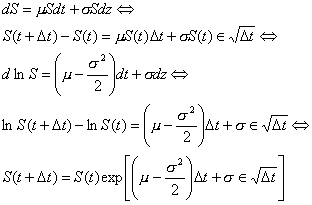We are coming to the final stage by using the rule: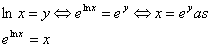So,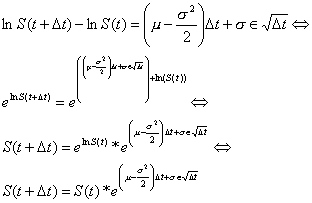By simply implementing the above formulae and solving to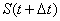we can get the evolution of the stock. The method that is regularly used is to create several paths for the stock and get an average of the price at the last day. An advantage of the MC is that it can be used when the payoff depends on the path followed by the underlying variable S, as well as when it depends only on the final value of S. there are many ways you can get random numbers to use in the MC. One is simply to use the Random Number Generator in Excel which gives you the ability to download random numbers according to the distribution you want these data to follow. Another way of getting variables from a normal distribution is by implementing the following equation:.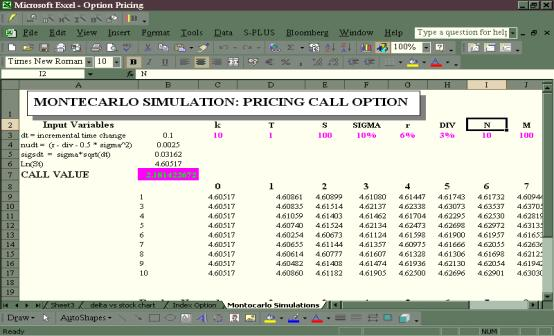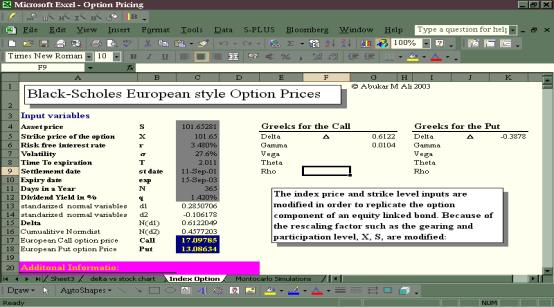In additional to using the numerical Monte Carlo pricing technique for path dependent, American, plain vanilla and other more exotic type of options, a European style option can also be priced by using the Black-Scholes pricing framework.

As the screen shows, to price the latter option, one can see all the required parameters to price the option and the cells from C4 to C12 can be used to input the required parameters. The result output for both the call and the put for the same underling can be observed from cell 17 and 18.

As an exercise change the input parameters and see the impact of the option premium with respect to these changes.  In fact, one can use this pricing technique to price similar option that is also attached to other assets such as capital guaranteed products.

For detailed discussion on this see the article by  Abukar M Ali, "Equity Linked Notes: and Introduction to Principal Guaranteed Structures",  Journal of Bond Trading & Management, Volume 1, Number 3, January 2003.

Home   What's New   Market Research   Presentations
RATE yield curve model   Excel Spreadsheets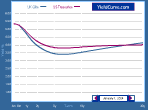© YieldCurve.comDisclaimerweb design